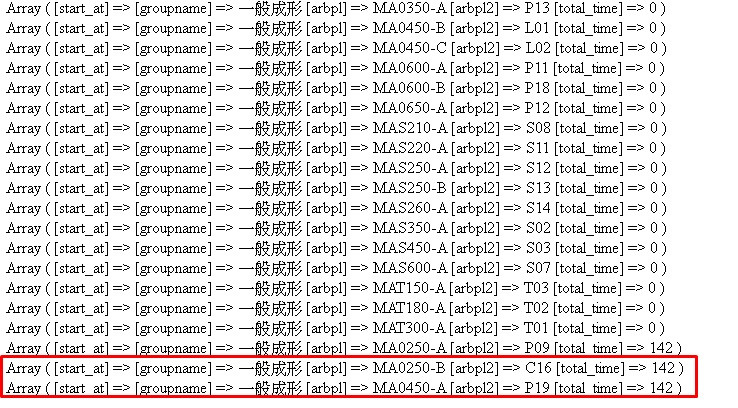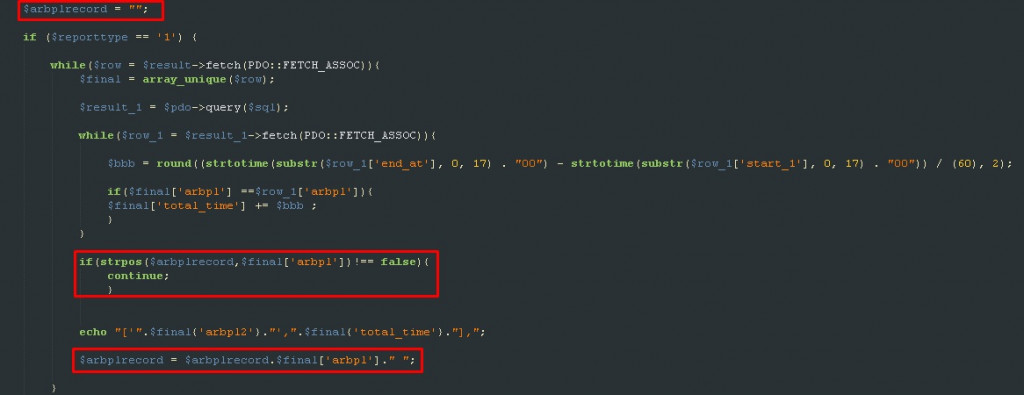#0

## <已解決>php - array_unique 排序問題1.asort(\$array);
2.array_multisort(\$array);

``````<?php

while(\$row = \$result->fetch(PDO::FETCH_ASSOC)){
\$final = array_unique(\$row);

\$result_1 = \$pdo->query(\$sql);

while(\$row_1 = \$result_1->fetch(PDO::FETCH_ASSOC)){

\$bbb = round((strtotime(substr(\$row_1['end_at'], 0, 17) . "00") - strtotime(substr(\$row_1['start_1'], 0, 17) . "00")) / (60), 2);

if(\$final['arbpl'] ==\$row_1['arbpl']){
\$final['total_time'] += \$bbb ;
}
}

if(\$final['arbpl']=='' and \$final['total_time']==''){
continue;
}

if(\$final['total_time']==''){
\$final['total_time'] === 0;
}

echo "['".\$final{'arbpl2'}."',".\$final{'total_time'}."],";

}
?>
``````### 2 個回答

1

s1031532 iT邦新手 5 級 ‧ 2020-07-27 10:35:13 檢舉

fillano iT邦超人 1 級 ‧ 2020-07-28 09:25:50 檢舉

1